## SATqPCR FAQ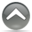#### How to design an experiment ?

You should at least have 3 biological replicates and at least 2 technical replicates for each sample of your experiments. Each gene you want to quantify should have a validated essay according to the MIQE standard. To follow the MIQE you should have calculated the efficiency of the primers you used to amplify a given sequence. This validation is done using calibration curves with at least 5 dilution points. The efficiency of the PCR reaction should be between 90-100% with an R2 between 0.99-1.
Amplicons of different genes can have different efficiency values but their all should be in the range of 90-100%. Once you have validated your amplicon for each gene of your assay you can use them to estimate the expression value of these genes in our experiments. SATqPCR is taking into account the efficiency difference between your GOI. In the text file you provide for the analysis (example.txt) you have to give the efficiency for each gene amplification in the first row.
The design of your experiment is describing the number of biological samples including your biological control, the number of technical replicates, and the number of genes tested (GOI and putative reference genes).#### How to enter your data

Input data must have the structure of our example file "example.txt".
- samplename_X where X is the number identifying the biological replicate
- all technical replicates of the same biological sample have exactly the same name
- All samples from the same biological replicate have to be in a same block,
each block corresponds to a biological replicate i (i=1,... m, "sample1_i...samplei_i"

This is represented in the table below :
``````Sample		GENE1	GENE2	GENE3	GENE1	GENE2	GENE3	 <= one column by gene
efficiency 	1.96	1.77	2.2	1.93	1.83	1.87
sample1_1	30.5	30.83	32.77	18.92	32.48	18.37	|
sample1_1	31.08	30.83	33.03	19.46	33.67	19.72	|
sample2_1	30.12	29.76	32.95	15.73	32.9	18.91	| Biological replicate 1
sample2_1	30.44	30.08	32.45	15.82	32.27	18.91	|
sampleN_1	30.68	30.37	33.24	17.56	31.87	20.16	|
sampleN_1	31.63	30.73	33.64	17.21	32.46	20.01	|
sample1_2	30.5	29.11	31.62	19.84	34.49	27.22	|
sample1_2	30	29.19	32.08	19.74	32.56	27.11	|
sample2_2	30.97	30.15	33.4	18.09	35.13	28.28	| Biological replicate 2
sample2_2	30.89	30.04	33.73	17.4	34.86	28.25	|
sampleN_2	31.56	30.5	34.2	18.95	32.53	23.53	|
sampleN_2	31.48	30.88	33.9	18.16	32.11	23.32	|
sample1_m	31.07	30.41	32.56	22.11	34.66	30.02	|
sample1_m	31.84	30.84	33.98	21.91	34.67	29.94	|
sample2_m	31.27	30.5	33.16	17.91	32.98	28.44	| Biological replicate m
sample2_m	31.77	30.73	33.72	17.63	33.92	28.17	|
sampleN_m	31.5	31.77	34.51	19.61	33.82	29.42	|
sampleN_m	32.43	32	34.33	19.01	32.68	29.3	|``````
```The missing values must be replaced by 'NA' value.
The number of technical replications must be identical across all samples.
The missing technical replication of a given sample should be added by a row where all values are 'NA' value.
Your sample names and your gene names should not have a “space” or “.” or “,” or “;” or “\$” or “\” or “/”.
Your data should be in a text file with a tabular separator.```#### What are REF genes ?

REFs are the most stably expressed genes across all tested samples and all biological replicates of one data set. Their identification is based on the stability parameter and coefficient of variation. SATqPCR uses the algorithm described in (Vandesompele et al. Genome Biology (2002) 3 (7):0034 1-11 ) to identify the reference genes. The REFs are specific to your studies and should be validated for each data set you want to analyze.
You can provide a list of reference genes by yourself but we DO NOT RECOMMEND it. Otherwise, SATqPCR will provide a list of reference genes for you by finding the most stables genes (at least 2).#### Statistical notation

``````m	number of biological replicates (index i)
n	number of technical replicates (index j)
r	number of reference genes (index ??)
Ej	PCR amplification efficiency coefficient of gene j
k	number of samples
Cq	quantitative cycle
QCq	relative quantity
SD	standard deviation
SE	standard error
NF	normalization factor
M	degree of freedom``````#### Computation of normalization factor ?

The normalization factor of sample k in biological replicate i across r reference genes, noted NFik, is given by the geometric mean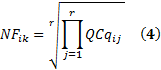And the standard deviation of this normalization factor is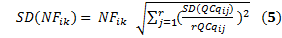with r corresponding to the number of reference genes.#### Normalization of relative quantities

For each biological replicate i,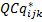is the normalization of relative quantity of GOI j for each sample k and is calculated by dividing the raw GOI quantity (2) by the normalization factor (4) as follows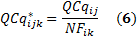and the standard deviation of this normalized GOI expression is given by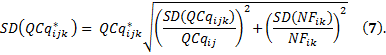The standard error quantifies scatter. To assess the precision of the mean calculation, we also provide the standard error (SE) value. The confidence interval defined by the measured mean ±1.96 times the SE has a 95% chance of containing the true mean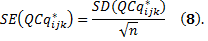Now, the average of expression level of GOI j for each sample k across m biological replicates is calculated by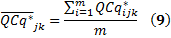and the SD and SE are given by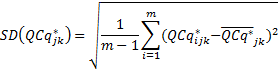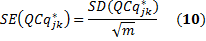Finally, the user can use the reference sample k,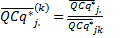or the smallest sample for rescaling the expression level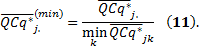The SD and SE corresponding to the transformation in (11) are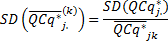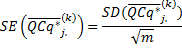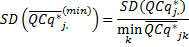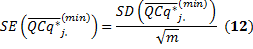#### Computation of relative quantities

For each biological replicate i, the average of Cq is computed for n technical replicates of the same gene j: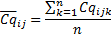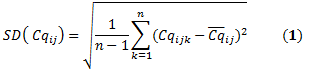The relative quantity associated with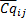is expressed as follows where the highest expression level set to one,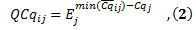and the standard deviation of QCqij is obtained from (2):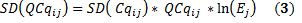#### How do we compare samples ?

Comparison between samples is performed using Statistical Significant Test. The user can performed either a t-test or an ANOVA.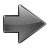t-test
For each GOI, all pairs of samples across all biological replicates are compared. Here we used the classical method of pairwise t-tests with a pooled standard deviation. We assumed that the two sample sizes are equal and the two distributions have the same variance. In order to apply the t-test, we have to ensure that the test samples follow Gaussian distributions. Here we use a simple logarithm transformation to transform the normalized relative expression,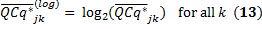Now, the t statistic to test whether the means are different between the samples S_i and S_j follows a Student's t-distribution with M-1 degrees of freedom and can be calculated as follows: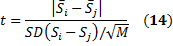where SD(Si-Sj) is the standard deviation of the differences between the Si and the Sj:
Once a t value is determined, the p-value of the test can be calculated from Student's t-distribution with M-1 degrees of freedom. The user can choose a threshold for the statistical significance to reject or accept the null hypothesis (H0: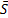i=j) in favor of the alternative hypothesis (Ha:i≠j).ANOVA
You should give the ANOVA factors associated to your experimental design in a text file. See factor.txt example.
Assumption: normal distributions and independence of the observations
Total Variability= variability explained by the factors + residual variability

The factor.txt file should contain the different factors associated for each sample :

``````Sample	Factor1	Factor2	Factor3	FactorN	  <= one column by factor
sample1	info1	info2	info3	infoN	|
sample2	info1	info2	info3	infoN	| <= one row by sample
sampleN	info1	info2	info3	infoN	|``````
``````Sample	Genotypes  Time  Tissues
col0h1	Columbia0  1h	 leaf
col2h1	Columbia0  2h	 leaf
col4h1	Columbia0  3h	 leaf
M0h1	Mutant1	   1h	 leaf
M2h1	Mutant1	   2h	 leaf
M4h1	Mutant1	   3h	 leaf``````
Factor1, Genotypes, has two modalities: Columbia0 and Mutant1.
Factor2, Time Course, has 3 modalities 1h, 2h and 4h.
Factor3, Tissues, has 2 modalities: tissues and leaf. Etc.#### How to rescale the normalized expression for each GOI

##### Rescaling by minimal sample value
By default, for each gene, your data are rescaled using the sample with the lowest expression.

##### Rescaling by a specific sample
You can rescale your expression data to a chosen sample. You will have to choose the sample name in the box "Sample name used to rescal"#### Graphical representation of your analysis

SATqPCR will plot rescaled expression for each GOI:
• By default, SATqPCR plots all genes and all samples. We will have a figure per gene (all the samples expression values for one gene) and a figure per sample (all the gene expression values for one sample).
• You can select the genes you want to plot across all samples
• You can select the samples you want to plot across all genes
• You can plot the gene expressions with either their standard deviations or with their standard errors (SE). Remember: There is a 95% chance that the confidence interval defined by the measured mean ±1.96 times the SE contains the true mean.#### Result description

##### SATqPCR returns the following TABLES
'rescaled.expression.txt': all GOI rescaled expression values
'sd.rescaled.expression.txt': standard deviations for all GOI rescaled expression
'se.rescaled.expression.txt': standard errors for all GOI rescaled expression.
'sign.test.gene_GOI': result of the t-test between samples for a GOI. SATqPCR returns for each GOI a sign.test.gene_GOI table.

##### SATqPCR returns the following plot for each GOI and each sample
Gene_GOI: normalized expression level with either SD or SE for all samples
Sample_samplei: normalized expression level with either SD or SE for all GOIs

##### SATqPCR returns for one-way ANOVA: aov_GOI_factorx
 Factor1 residual Sum of Squares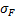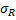Deg. of Freedom p-1 n-p Residual standard error: #
p : number of modality for factor1
n : number of samplei
##### SATqPCR returns for two-way ANOVA: aov_GOI_factorx
 Factor1 Factor2 residual Sum of Squares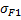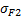Deg. of Freedom p1-1 p2-1 n-p1-p2 Residual standard error: #
p1 : number of modalities for factor1
p2 : number of modalities for factor2
n : number of samplei
##### SATqPCR returns for one-way ANOVA or two-way ANOVAtukeyTEST_GOI_factorx

For each factor SATqPCR returns a table as follow

 diff lwr upr padj B-A -0.06666667 -1.803101 1.669768 0.9950379 C-A -3.80000000 -5.536435 -2.063565 0.0000260 C-B -3.73333333 -5.469768 -1.996899 0.0000337
diff : difference between the estimated means of compared modalities
lwr : lower bound of the confidence interval estimated for diff
upr : upper bound of the confidence interval estimated for diff
padj : p value for multiple comparisons (Tukey's Honest Significant Difference method)
A, B, C are all the modalities of factorx.
This output indicates that the differences C-A and C-B are significant, while B-A is not significantNormalized_Data_GOI

One for each GOI

 Samples factorx Replicat Value Sample1 A 1 Sample1 B 1 Sample2 A 2 Sample2 B 2 Samplei # #
A and B are the modalities of factorx, Replicat identifies the biological replicate and Value is the normalized gene expression value of GOI

##### SATqPCR returns the following plot for each GOI and each sample
- Gene_GOI: normalized expression level with either SD or SE for all samples
- Sample_samplei: normalized expression level with either SD or SE for all GOIs